Most Affordable JEE | NEET | 8,9,10 Preparation by Kota's Top IITian Doctor Faculties

# NCERT Solutions for Class 11 Chemistry Chapter 1 Some Basic Concepts of Chemistry - Free PDF download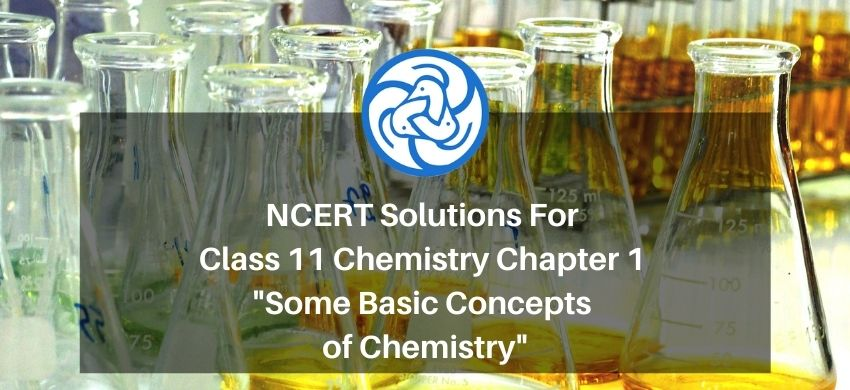`
Hey, are you a class 11 student and looking for ways to download NCERT Solutions for Class 11 Chemistry Chapter 1 Some Basic Concepts of Chemistry PDF? If yes. Then read this post till the end.

In this article, we have listed NCERT Solutions for Class 11 Chemistry Chapter 1 Some Basic Concepts of Chemistry in PDF that are prepared by Kota’s top IITian’s Faculties by keeping Simplicity in mind.

If you want to learn and understand class 11 Chemistry chapter 1 "Some Basic Concepts of Chemistry" in an easy way then you can use these solutions PDF.

NCERT Solutions helps students to Practice important concepts of subjects easily. Class 11 Chemistry solutions provide detailed explanations of all the NCERT questions that students can use to clear their doubts instantly.

If you want to score high in your class 11 Chemistry Exam then it is very important for you to have a good knowledge of all the important topics, so to learn and practice those topics you can use eSaral NCERT Solutions.

In this article, we have listed NCERT Solutions for Class 11 Chemistry Chapter 1 Some Basic Concepts of Chemistry that you can download to start your preparations anytime.

So, without wasting more time Let’s start.

### Download NCERT Solutions for Class 11 Chemistry Chapter 1 Some Basic Concepts of Chemistry PDF"

Question 1. Calculate the molar mass of the following:

(i) $\mathrm{H}_{2} \mathrm{O}$
(ii) $\mathrm{CO}_{2}$

(iii) $\mathrm{CH}_{4} \mathrm{~S}$

Solution: (i) The molecular mass of water $\left(\mathrm{H}_{2} \mathrm{O}\right)$

We know : Atomic mass of hydrogen $=1.008 \mathrm{u}$

Atomic mass of oxygen $=16.00 \mathrm{u}$

molecular mass of $\mathrm{H}_{2} \mathrm{O}=(2 \times$ Atomic mass of hydrogen $)$

$+(1 \times$ Atomic mass of oxygen)

$=[2(1.008 \mathrm{u})+1(16.00 \mathrm{u})]$

$=2.016 \mathrm{u}+16.00 \mathrm{u}$

$=18.016 \mathrm{u}$

(ii) The molecular mass of carbon dioxide $\left(\mathrm{CO}_{2}\right)$

Atomic mass of carbon $=12.0 \mathrm{u}$

Atomic mass of oxygen $=16.00 \mathrm{u}$

$=(1 \times$ Atomic mass of carbon $)$

$+(2 \times$ Atomic mass of oxygen $)$

$=[1(12.0 \mathrm{u})+2(16.00 \mathrm{u})]$

$=12.0 \mathrm{u}+32.00 \mathrm{u}$

$=44.0 \mathrm{u}$

(iii) The molecular mass of methane $\left(\mathrm{CH}_{4}\right)$

Atomic mass of carbon $=12.0 \mathrm{u}$

Atomic mass of hydrogen $=1.008 \mathrm{u}$

$=(1 \times$ Atomic mass of carbon $)$

$+(4 \times$ Atomic mass of hydrogen $)$

$=[1(12.0 \mathrm{u})+4(1.008 \mathrm{u})]$

$=12.0 \mathrm{u}+4.032 \mathrm{u}$

$=16.032 \mathrm{u}$

Question 2. Calculate the mass percent of different elements present in sodium sulphate $\left(\mathrm{Na}_{2} \mathrm{SO}_{4}\right)$.

Solution.We know: Atomic mass of sodium $=23.0 \mathrm{u}$

Atomic mass of Sulphur $=32.0 \mathrm{u}$

Atomic mass of carbon $=16.00 \mathrm{u}$

Calculate : The molecular formula of sodium sulphate is $\mathrm{Na}_{2} \mathrm{SO}_{4}$

Molar mass of $\mathrm{Na}_{2} \mathrm{SO}_{4}$

$=[(2 \times 23.0)+(32.0)+4(16.00)]$

$=142 \mathrm{u}$ or $142 \mathrm{~g}$

Formula: Mass percent of an element

$=\frac{\text { Mass of that element in the compound }}{\text { Molar mass of the compound }} \times 100$

$\therefore$ Mass percent of sodium

$==\frac{\text { Mass of sodium element in the compound }}{\text { Molar mass of the } \mathrm{Na}_{2} \mathrm{SO}_{4}} \times 100$

$=\frac{46.0 \mathrm{~g}}{142.0 \mathrm{~g}} \times 100$

$=32.39$

$=32.4 \%$

Mass percent of Sulphur

$=\frac{\text { Mass of sulphur element in the compound }}{\text { Molar mass of the } \mathrm{Na}_{2} \mathrm{SO}_{4}} \times 100$

$=\frac{32.0 \mathrm{~g}}{142.0 \mathrm{~g}} \times 100$

$=22.54 \%$

Mass percent of oxygen

$=\frac{\text { Mass of oxygen element in the compound }}{\text { Molar mass of the } \mathrm{Na}_{2} \mathrm{SO}_{4}} \times 100$

$=\frac{64.0 \mathrm{~g}}{142.0 \mathrm{~g}} \times 100$

$=45.07 \%$

Question 3. Determine the empirical formula of an oxide of iron, which has $69.9 \%$ iron and $30.1 \%$ dioxygen by mass.

Solution: Given : $\%$ of iron by mass $=69.9 \%$

$\%$ of oxygen by mass $=30.1 \%$

Atomic mass of iron $=55.85$

Atomic mass of oxygen $=16.00$

Formula used= Relative moles of atom in compound

$=\frac{\% \text { by mass of atom }}{\text { Atomic mass of atom }}$

Relative moles of iron in iron oxide

$=\frac{\% \text { by mass of iron }}{\text { Atomic mass of iron }}$

$=\frac{69.9}{55.85}$

$=1.25$

Relative moles of oxygen in iron oxide:

$=\frac{\% \text { of oxygen by mass }}{\text { Atomic mass of oxygen }}$

$=\frac{30.1}{16.00}$

$=1.88$

Simplest molar ratio of iron to oxygen:

$=1.25: 1.88$

$=1: 1.5$

$=2: 3$

$\therefore$ The empirical formula of the iron oxide is $\mathrm{Fe}_{2} \mathrm{O}_{3}$

Question 4. Calculate the amount of carbon dioxide that could be produced when

(i) 1 mole of carbon is burnt in air.

(ii) 1 mole of carbon is burnt in $16 \mathrm{~g}$ of dioxygen.

(iii) 2 moles of carbon is burnt in $16 \mathrm{~g}$ of dioxygen.

Solution. The balanced reaction of combustion of carbon can be written as:

(i)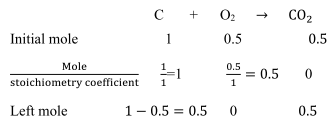As per stoichiometry of the balanced equation, 1 mole of carbon burns in 1 mole of dioxygen (air) to Produced 1 mole of carbon dioxide.

(ii) 1 mole of carbon is burnt in $16 \mathrm{~g}$ of dioxygen.

Given in question: dioxygen $=16 \mathrm{~g} ;$ mole of carbon $=1$

We know : Moles of oxygen

$=\frac{\text { moles }}{\text { molecular weight }}=\frac{16}{32}=0.5$According to the stoichiometry of the balanced equation

Hence, dioxygen will react with $0.5$ moles of carbon to give $0.5$ mole of carbon dioxide

( $22 \mathrm{~g}$ ). Hence, dioxygen is a limiting reactant.

(iii) $\mathrm{C}+\mathrm{O}_{2} \rightarrow \mathrm{CO}_{2}$

Given : dioxygen $\left(O_{2}\right)=16 \mathrm{~g} \quad ;$ mole of carbon $=2$

We know : molecular weigth of $\mathrm{O}_{2}=32$

moles of dioxygen $=\frac{\text { weight }}{\text { molecular weight }}=\frac{16}{32}=0.5$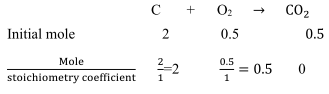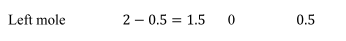According to the question, only $0.5$ mole of dioxygen or is available. It is a limiting

reactant. Thus, $0.5$ mole of dioxygen can combine with only $0.5$ mole of carbon dioxide

Weight of carbon dioxide $=$ moles $\times$ molecular weight of $\mathrm{CO}_{2}=0.5 \times 44=22$ gram

Question 5. Calculate the mass of sodium acetate $\left(\mathrm{CH}_{3} \mathrm{COONa}\right)$ required to make $500 \mathrm{~mL}$ of $0.375$molar aqueous solution. Molar mass of sodium acetate is $82.0245 \mathrm{gmol}^{-1}$.

Solution: given : mass of sodium acetate $\left(\mathrm{CH}_{3} \mathrm{COONa}\right)=?$

Molar mass of sodium acetate $=82.024 \mathrm{gmole}^{-1}$

Molarity of aqueous solution of sodium acetate $=0.375 \mathrm{M}$

Volume of solution $=500 \mathrm{ml}$

we know : $\therefore$ A number of moles of sodium acetate in $500 \mathrm{~mL}$.

$=$ Molarity $\times$ volume of

solution (litre)

$=\frac{0.375}{1000} \times 500$

$=0.1875$ mole

$\therefore$ Required mass of sodium acetate $=$ moles $\times$ molecular weight

$\Rightarrow\left(82.0245 \mathrm{gmo} 1^{-1}\right) \times(0.1875 \mathrm{~mole})$

$\Rightarrow 15.38 \mathrm{~g}$

Question 6. Calculate the concentration of nitric acid in moles per liter in a sample which has adensity, $1.41 \mathrm{gmL}^{-1}$ and the mass percent of nitric acid in it being $69 \%$.

Solution: Given : density $=1.41 \mathrm{gmL}^{-1}$

Mass percent of nitric acid in the sample $=69 \%$

We know, the mass percent of nitric acid in it being $69 \%=100 \mathrm{~g}$ of nitric acid contains

$69 \mathrm{~g}$ of nitric acid by mass.

Molar mass of nitric acid $\left(\mathrm{HNO}_{3}\right)$

$=\{1+14+3(16)\} \mathrm{gmo} 1^{-1}$

$=(1+14+48) \mathrm{gmo} 1^{-1}$

$=63 \mathrm{gmo} 1^{-1}$

$\therefore$ Number of moles in $69 \mathrm{~g}$ of $\mathrm{HNO}_{3}$

$=\frac{\text { weight }(\mathrm{g})}{\text { molecular mass }\left(\mathrm{gmo} 1^{-1}\right)}$

$=\frac{69 \mathrm{~g}}{63 \mathrm{gmo} 1^{-1}}$

Volume of $100 \mathrm{~g}$ nitric acid solution

$=\frac{\text { Mass of solution }}{\text { density of solution }}$

$=\frac{100 \mathrm{~g}}{1.41 \mathrm{gmL}^{-1}}$

$=\frac{100 \mathrm{~g}}{141 \mathrm{gmL}^{-1}} \times 100$

$=70.92 \mathrm{~mL} \equiv 70.92 \times 10^{-3} \mathrm{~L}$

$\left(\therefore 1 \mathrm{ml}=10^{-3}\right.$ liter $)$ )

Concentration of nitric acid $=\frac{\text { mole }}{\text { litre }}$

$=\frac{1.095 \mathrm{mo} 1 \mathrm{e}}{70.92 \times 10^{-3} \mathrm{~L}}$

$=15.44 \mathrm{~mol} / \mathrm{L}$

$\therefore$ Concentration of nitric acid $=15.44 \mathrm{~mol} / \mathrm{L}$

Question 7. How much copper can be obtained from $100 \mathrm{~g}$ of copper sulphate $\left(\mathrm{CuSO}_{4}\right)$ ?

Solution: Given : mass of $\mathrm{CuSO}_{4}=100 \mathrm{~g}$

We know : Molar mass of $\mathrm{CuSO}_{4}$

$=(63.5)+(3200)+4(1600)$

$=63.5+32.00+64.00$

$=159.5 \mathrm{~g}$

$159.5 \mathrm{~g}$ of $\mathrm{CuSO}_{4}$ contains $63.5 \mathrm{~g}$ of copper.

$\Rightarrow 100 \mathrm{~g}$ of $\mathrm{CuSO}_{4}$ will contain copper
$=\frac{63.5 \times 100 \mathrm{~g}}{159.5}=39.81 \mathrm{~g}$

$\therefore$ Amount of copper that can be obtained from $100 \mathrm{~g} \mathrm{CuSO}_{4}=39.81 \mathrm{~g}$

Question 8. Determine the molecular formula of an oxide of iron, in which the mass percent of iron and oxygen are $69.9$ and $30.1$, respectively.

Solution: Given : Mass percent of iron (Fe) $=69.9 \%$

Mass percent of oxygen $(0)=30.1 \%$

We know: The atomic mass of iron $=55.85$

The atomic mass of oxygen $=16$

Number of moles of iron present in the oxide

$=\frac{69.90}{55.85}=1.25$

Number of moles of oxygen present in the oxide

$=\frac{30.1}{16.0}=1.88$

The ratio of iron to oxygen in the oxide,

$=1.25: 1.88$

$=\frac{1.25}{1.25}: \frac{1.88}{1.25}$

$=1: 1.5$

$=2: 3$

$\therefore$ The empirical formula of the oxide is $\mathrm{Fe}_{2} \mathrm{O}_{3}$

Empirical formula mass of $\mathrm{Fe}_{2} \mathrm{O}_{3}$

$=[2(55.85)+3(16.00)] g$

Molar mass of $\mathrm{Fe}_{2} \mathrm{O}_{3}=159.69 \mathrm{~g}$

$\therefore \mathrm{n}=\frac{\text { Molar mass }}{\text { Empirical formula mass }}=\frac{159.69 \mathrm{~g}}{159.7 \mathrm{~g}}$

$=0.999$

$=1($ approx $)$

Molecular formula of a compound

$=$ (empirical formula of a compound) $\mathrm{n}$.

Thus, molecular formula $=\left(\mathrm{Fe}_{2} \mathrm{O}_{3}\right) \times 1$

Hence, the molecular formula of the oxide is $\mathrm{Fe}_{2} \mathrm{O}_{3}$.

Question 9. Calculate the atomic mass (average) of chlorine using the following data: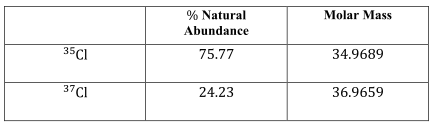Solution: given in question :

for ${ }^{35} \mathrm{Cl}: \%$ Natural Abundance $=75.77$

Molar Mass $=34.9689$

${ }^{37} \mathrm{Cl}: \%$ Natural Abundance $=24.23$

Molar Mass $=36.9659$

The average atomic mass of chlorine.

$=\left[\left(\begin{array}{c}\text { Fractional abundance } \\ \text { of }^{35} \mathrm{Cl}\end{array}\right)\left(\begin{array}{c}\text { Molar mass } \\ \text { of }^{35} \mathrm{Cl}\end{array}\right)\right.$

$\left.+\left(\begin{array}{c}\text { Fractional abundance } \\ \text { of }^{37} \mathrm{Cl}\end{array}\right)\left(\begin{array}{c}\text { Molar mass } \\ \text { of }^{37} \mathrm{Cl}\end{array}\right)\right]$

$=\left[\left\{\left(\frac{75.77}{100}\right)(34.9689 \mathrm{u})\right\}\right.$

$\left.+\left\{\left(\frac{24.23}{100}\right)(36.9659 \mathrm{u})\right\}\right]$

$=26.4959 \mathrm{u}+8.9568 \mathrm{u}$

$=35.4527 \mathrm{u}$

$\therefore$ The average atomic mass of chlorine $=35.4527 \mathrm{u}$

Question 10. In three moles of ethane $\left(\mathrm{C}_{2} \mathrm{H}_{6}\right)$, calculate the following:

(i) Number of moles of carbon atoms.

(ii) Number of moles of hydrogen atoms.

(iii) Number of molecules of ethane.

Solution: (i) 1 mole of $\mathrm{C}_{2} \mathrm{H}_{6}$ contains 2 moles of carbon atoms.

$\therefore$ Number of moles of carbon atoms in 3 moles of $\mathrm{C}_{2} \mathrm{H}_{6}$

$=2 \times 3=6$ moles of carbon

(ii) 1 mole of $\mathrm{C}_{2} \mathrm{H}_{6}$ contains 6 moles of hydrogen atoms.

$\therefore$ Number of moles of carbon atoms in 3 moles of $\mathrm{C}_{2} \mathrm{H}_{6}$

$=3 \times 6=18$ moles of hydrogen atoms

(iii) 1 mole of $\mathrm{C}_{2} \mathrm{H}_{6}$ contains $=\mathrm{N}_{\mathrm{A}} \times$ molecules of ethane

$=6.023 \times 10^{23} \times$ molecules of ethane.

$\therefore$ Number of molecules in 3 moles of

$\mathrm{C}_{2} \mathrm{H}_{6}=3 \times \mathrm{N}_{\mathrm{A}}=3 \times 6.023 \times 10^{23}$

$=18.069 \times 10^{23}$ molecules of ethane

Question 11. What is the concentration of sugar $\left(\mathrm{C}_{12} \mathrm{H}_{22} \mathrm{O}_{11}\right)$ in $\mathrm{molL}^{-1}$ if its $20 \mathrm{~g}$ are dissolved in

enough water to make a final volume up to $2 \mathrm{~L}$ ?

Solution: Given mass of glucose $=20 \mathrm{~g}$

We know : molar mass of sugar $\left(\mathrm{C}_{12} \mathrm{H}_{22} \mathrm{O}_{11}\right)$

$=[(12 \times 12)+(1 \times 22)+(11 \times 16)]=$

342

Formula: Molarity of a solution (M)

$=\frac{\text { Number of moles of solute }}{\text { Volume of solution in Litres }}$

By putting the values in formula we get

$\mathrm{M}=\frac{\text { Mass of sugar/molar mass of sugar }}{2 \mathrm{~L}}$

$=\frac{20 g / 342}{2 L}$

$=\frac{0.0585 \mathrm{~mol}}{2 \mathrm{~L}}$

$=0.02925 \mathrm{molL}^{-1}$

$\therefore$ Molar concentration of sugar $=0.02925 \mathrm{molL}^{-1}$

Question 12. If the density of methanol is $0.793 \mathrm{kgL}^{-1}$ what is its volume needed for making $2.5 \mathrm{~L}$ of

its $0.25 \mathrm{M}$ solution?

Solution: Given:

density of methanol is $=0.793 \mathrm{kgL}^{-1}$

volume of solution $\left(\mathrm{V}_{2}\right)=2.5 \mathrm{~L}$

molarity of solution $\left(\mathrm{M}_{2}\right)=0.25 \mathrm{M}$

we know Molar mass of methanol $\left(\mathrm{CH}_{3} \mathrm{OH}\right)$

$=(1 \times 12)+(4 \times 1)+(1 \times 16)=$

$32 \mathrm{gmo} 1^{-1}=0.032 \mathrm{kgmol}^{-1}$

density of methanol is $0.793 \mathrm{kgL}^{-1}$ means one litre of solution contain

$0.793 \mathrm{~kg}$ of methanol $=793 \mathrm{gm}$ of methanol

$=\frac{793}{32}$ moles of methanol

i. e. molarity of methanol volume $\left(M_{1}\right)=24.78 \mathrm{~mol} \mathrm{~L}^{-1}$

formula used : $\mathrm{M}_{1} \mathrm{~V}_{1}=\mathrm{M}_{2} \mathrm{~V}_{2}$

$\left(24.78 \mathrm{molL}^{-1}\right) \mathrm{V}_{1}=(2.5 \mathrm{~L})\left(0.25 \mathrm{~mol} \mathrm{~L}^{-1}\right)$

$\mathrm{V}_{1}=0.0252 \mathrm{~L}$

(1 liter= 1000 mililiter)

$\mathrm{V}_{1}=25.22 \mathrm{~mL}$

Question 13. Pressure is determined as force per unit area of the surface. The Sl unit of pressure, pascal is as shown below:

$1 \mathrm{~Pa}=1 \mathrm{Nm}^{-2}$

If the mass of air at sea level is $1034 \mathrm{gcm}^{-2}$ calculate the pressure in Pascal.

Solution: Given : mass of air at sea level $=1034 \mathrm{gcm}^{-2}$

The pressure is defined as force acting per unit area of the surface.

$P=\frac{F}{A}$

$=\frac{1034 \mathrm{~g} \times 9.8 \mathrm{~ms}^{-2}}{\mathrm{~cm}^{2}} \times \frac{1 \mathrm{~kg}}{1000 \mathrm{~g}}$

$\times \frac{(100)^{2} \mathrm{~cm}^{2}}{1 \mathrm{~m}^{2}}$

$=1.01332 \times 10^{5} \mathrm{kgm}^{-1} \mathrm{~s}^{-2}$

We know,

$1 \mathrm{~N}=1 \mathrm{kgms}^{-2}$

Then,

$1 \mathrm{~Pa}=1 \mathrm{Nm}^{-2}=1 \mathrm{kgm}^{-2} \mathrm{~s}^{-2}$

$1 \mathrm{~Pa}=1 \mathrm{kgm}^{-1} \mathrm{~s}^{-2}$

$\therefore$ Pressure $=1.01332 \times 10^{5} \mathrm{~Pa}$

Question 14. What is the SI unit of mass? How is it defined?

Solution: The SI unit of mass is a kilogram (kg).

1 kilogram is defined as the mass equal to the mass of the international

prototype(Platinum-Iridium cylinder in Paris) of the kilogram.

Question 15. Match the following prefixes with their multiples: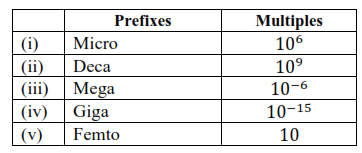Solution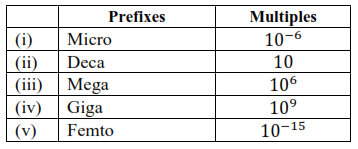Question 16. What do you mean by significant figures?

Solution. Significant figures are those meaningful digits that are known with certainty. They

indicate uncertainty in an experiment or calculated value. For example, if $25.8 \mathrm{~mL}$ is the

result of an experiment, then 25 is certain, while 8 is uncertain, and the total number of

significant figures are 3 . Hence, significant figures are defined as the total number of

digits in a number including the last digit that represents the uncertainty of the result.

Question 17. A sample of drinking water was found to be severely contaminated with chloroform
$\mathrm{CHCl}_{3}$ supposed to be carcinogenic in nature. The level of contamination was $15 \mathrm{ppm}$ (by mass).

(i) Express this in percent by mass.

(ii) Determine the molality of chloroform in the water sample.

Solution: (i) $1 \mathrm{ppm}=1$ part out of 1 million $\left(10^{6}\right)$ parts.

Let assume Mass of chloroform $\mathrm{CHCl}_{3}=15$

Mass of solution $=10^{6}$

Formula:

Mass \% of solute $=\frac{\text { mass of solute } \times 100}{\text { mass of solution }}$

$\therefore$ Mass percent of 15 ppm chloroform in water.

$=\frac{15}{10^{6}} \times 100$

$\approx 1.5 \times 10^{-3} \%$

(ii) $100 \mathrm{~g}$ of the sample contains $1.5 \times 10^{-3} \mathrm{~g}$ of $\mathrm{CHCl}_{3}$.

Given : weight of $\mathrm{CHCl}_{3}=1.5 \times 10^{-3} \mathrm{~g}$

Weight of solvent $=100 \mathrm{~g}$

We know : Molar mass of $\mathrm{CHCl}_{3}$

$=12.00+1.00+3(35.5)=119.5 \mathrm{gmo} 1^{-1}$

Formula : Molaity $=\frac{\frac{\text { weight of solute (gram) }}{\text { Molar mass of solute }}}{\text { weight of solvent }(\mathrm{kg})}$

Put all the above values in formula we get

Molality of chloroform in water.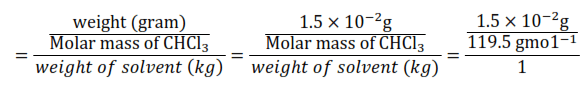$=0.0125 \times 10^{-2}$

$=1.25 \times 10^{-4} \mathrm{molal}$

Question 18. Express the following in the scientific notation:

(i) $0.0048$

(ii) 234,000

(iii) 8008

(iv) $500.0$

(v) $6.0012$

Solution: (i) $0.0048=4.8 \times 10^{-3}$

(ii) $234,000=2.34 \times 10^{5}$

(iii) $8008=8.008 \times 10^{3}$

(iv) $500.0=5.000 \times 10^{2}$

(v) $6.0012=6.0012$

Question 19. How many significant figures are present in the following?

(i) $0.0025$

(ii) 208

(iii) 5005

(iv) 126,000

(v) $500.0$

(vi) $2.0034$

Solution: (i) There are 2 significant figures.

(ii) There are 3 significant figures.

(iii) There are 4 significant figures.

(vi) There are 3 significant figures.

(v) There are 4 significant figures.

(vi) There are 5 significant figures.

Question 20. Round up the following upto three significant figures:

(i) $34.216$

(ii) $10.4107$

(iii) $0.04597$

(iv) 2808

Solution: (i) $34.2$

(ii) $10.4$

(iii) $0.0460$

(iv) 2810

Question 21. The following data are obtained when dinitrogen and dioxygen react together to form different compounds: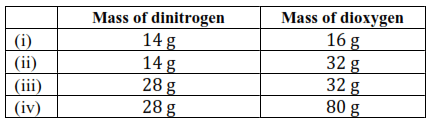(a) Which law of chemical combination is obeyed by the above experimental data? Give its statement.

(b) Fill in the blanks in the following conversions: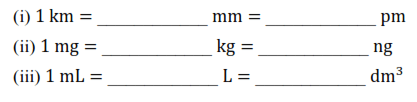Solution: (a) If we fix the mass of dinitrogen at $28 \mathrm{~g}$, then the masses of dioxygen that will combine

with the fixed mass of dinitrogen are $32 \mathrm{~g}, 64 \mathrm{~g}, 32 \mathrm{~g}$, and $80 \mathrm{~g}$.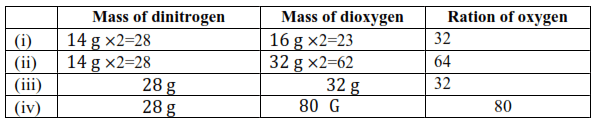The masses of dioxygen bear a whole number ratio of $1: 2: 1: 5 .$ Hence, the given

experimental data obeys the law of multiple proportions. The law states that if two elements

combine to form more than one compound, then the masses of one element that combines

with the fixed mass of another element are in the ration of small whole numbers.

(b) (i) $1 \mathrm{~km}=1 \mathrm{~km} \times \frac{1000 \mathrm{~m}}{1 \mathrm{~km}} \times \frac{100 \mathrm{~cm}}{1 \mathrm{~m}} \times \frac{10 \mathrm{~mm}}{1 \mathrm{~cm}}$

$\therefore 1 \mathrm{~km}=10^{6} \mathrm{~mm}$

$1 \mathrm{~km}=1 \mathrm{~km} \times \frac{1000 \mathrm{~m}}{1 \mathrm{~km}} \times \frac{1 \mathrm{pm}}{10^{-12} \mathrm{~m}}$

$\therefore 1 \mathrm{~km}=10^{15} \mathrm{pm}$

Hence, $1 \mathrm{~km}=10^{6} \mathrm{~mm}=10^{15} \mathrm{pm}$

(ii) $1 \mathrm{mg}=1 \mathrm{mg} \times \frac{1 \mathrm{~g}}{1000 \mathrm{mg}} \times \frac{1 \mathrm{~kg}}{1000 \mathrm{~g}}$

$\Rightarrow 1 \mathrm{mg}=10^{-6} \mathrm{~kg}$

$1 \mathrm{mg}=1 \mathrm{mg} \times \frac{1 \mathrm{~g}}{1000 \mathrm{mg}} \times \frac{1 \mathrm{ng}}{10^{-9} \mathrm{~g}}$

$\Rightarrow 1 \mathrm{mg}=10^{6} \mathrm{ng}$

$\therefore 1 \mathrm{mg}=10^{-6} \mathrm{~kg}=10^{6} \mathrm{ng}$

(iii) $1 \mathrm{~mL}=1 \mathrm{~mL} \times \frac{1 \mathrm{~L}}{1000 \mathrm{~mL}}$

$\Rightarrow 1 \mathrm{~mL}=10^{-3} \mathrm{~L}$

$1 \mathrm{~mL}=1 \mathrm{~cm}^{3}=1 \mathrm{~cm}^{3} \frac{1 \mathrm{dm} \times 1 \mathrm{dm} \times 1 \mathrm{dm}}{10 \mathrm{~cm} \times 10 \mathrm{~cm} \times 10 \mathrm{~cm}}$

$\Rightarrow 1 \mathrm{~mL}=10^{-3} \mathrm{dm}^{3}$

$\therefore 1 \mathrm{~mL}=10^{-3} \mathrm{~L}=10^{-3} \mathrm{dm}^{3}$

Question 22. If the speed of light is $3.0 \times 10^{8} \mathrm{~ms}^{-1}$ calculate the distance covered by light in $2.00 \mathrm{~ns}$.

Solution: Given : speed of light $=3.0 \times 10^{8} \mathrm{~ms}^{-1}$

distance covered by light $=?$

Time taken to cover the distance

$=2.00 \mathrm{~ns}=2.00 \times 10^{-9} \mathrm{~s}$

We know: velocity of light $=3.0 \times 10^{8} \mathrm{~ms}^{-1}$

Formula : Distance traveled by light

$=$ Speed of light $\times$ Time taken

$=\left(3.0 \times 10^{8} \mathrm{~ms}^{-1}\right)\left(2.00 \times 10^{-9} \mathrm{~s}\right)$

$=6.00 \times 10^{-1} \mathrm{~m}$

$=0.600 \mathrm{~m}$

Question 23. In a reaction $\mathrm{A}+\mathrm{B}_{2} \rightarrow \mathrm{AB}_{2}$

Identify the limiting reagent, if any, in the following reaction mixtures.

(i) 300 atoms of $A+200$ molecules of $B$

(ii) $2 \mathrm{~mol} \mathrm{~A}+3 \mathrm{~mol} \mathrm{~B}$

(iii) 100 atoms of $A+100$ molecules of $B$

(iv) $5 \mathrm{~mol} \mathrm{~A}+2.5 \mathrm{~mol} \mathrm{~B}$

(v) $2.5 \mathrm{~mol} A+5 \mathrm{~mol} \mathrm{~B}$

Solution. A reagent determines the extent of a reaction. It is the reactant which is the first to get

consumed during a reaction, thereby causing the reaction to stop and limiting the amount

of products formed.

(i) 300 atoms of $A+200$ molecules of $B$

According to the stoichiometry of reaction, 1 atom of $A$ reacts with 1 molecule of $B$.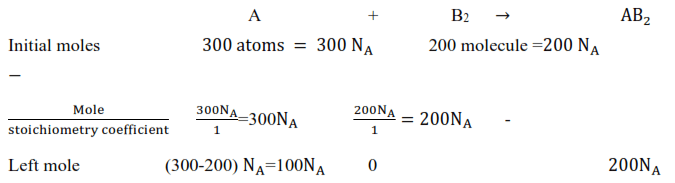Thus, $200 \mathrm{~N}_{\mathrm{A}}$ moles of $\mathrm{B}_{2}$ will react with $300 \mathrm{~N}_{\mathrm{A}}$ moles of $\mathrm{A}$, thereby leaving $100 \mathrm{~N}_{\mathrm{A}}$

moles of A unused. Hence, $\mathrm{B}_{2}$ is the limiting reagent.

(ii) $2 \mathrm{~mol} \mathrm{~A}+3 \mathrm{~mol} \mathrm{~B}$

According to the reaction, 1 mol of A reacts with 1 mol of 3 .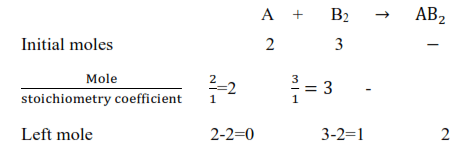Thus, 2 mol of $A$ will react with only 2 mol of $B$. As a result, 1 mol of A will not be

consumed. Hence, $\mathrm{A}$ is the limiting reagent.

(iii) 100 atoms of $\mathrm{A}+100$ molecules of $\mathrm{B}$

According to the given reaction, 1 atom of A combines with 1 molecule of $B$.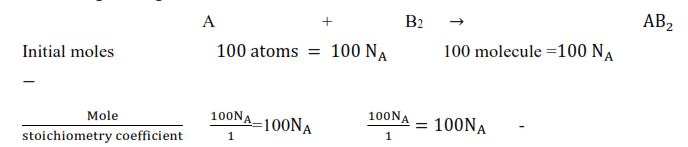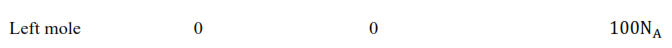Thus, all $100 \mathrm{~N}_{\mathrm{A}}$ moles of $\mathrm{A}$ will combine with all $100 \mathrm{~N}_{\mathrm{A}}$ moles of $\mathrm{B}$. Hence, the mixture

is stoichiometric where no limiting reagent is present.

(ii) $5 \mathrm{~mol} \mathrm{~A}+2.5 \mathrm{~mol} \mathrm{~B}$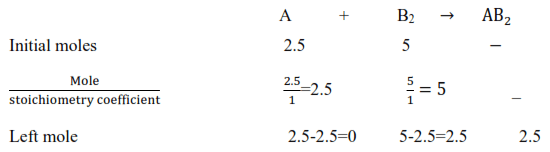According to the reaction, $1 \mathrm{~mol}$ of atom $\mathrm{A}$ combines with $1 \mathrm{~mol}$ of molecule $\mathrm{B}$. Thus,

$2.5 \mathrm{~mol}$ of $\mathrm{A}$ will combine with only $2.5 \mathrm{~mol}$ of $\mathrm{B}$ and the remaining $2.5 \mathrm{~mol}$ of $\mathrm{B}$ will be

left as such. Hence, $\mathrm{A}$ is the limiting reagent.

Question 24. Dinitrogen and dihydrogen react with each other to produce ammonia according to the following chemical reaction:

$\mathrm{N}_{2}(\mathrm{~g})+\mathrm{H}_{2}(\mathrm{~g}) \rightarrow 2 \mathrm{NH}_{3}(\mathrm{~g})$

(i) Calculate the mass of ammonia produced if $2.00 \times 10^{3} \mathrm{~g}$ dinitrogen reacts with

$1.00 \times 10^{3} \mathrm{~g}$ of dihydrogen.

(ii) Will any of the two reactants remain unreacted?

(iii) If yes, which one and what would be its mass?

Solution: Given : mass of dinitrogen $=2.00 \times 10^{3} \mathrm{~g}$

mass of dihydrogen $=1.00 \times 10^{3} \mathrm{~g}$

we should know : molecular weight of $\mathrm{N}_{2}=28$

molecular weight of $\mathrm{H}_{2}=2$

molecular weight of $\mathrm{NH}_{3}=34$

Moles of dinitrogen $=\frac{\text { weight }(g)}{\text { molecular weight }}$

$=\frac{2.00 \times 10^{3} \mathrm{~g}}{28}=71.43$

moles of dihydrogen $==\frac{\text { weight }(g)}{\text { molecular weight }}$

$=\frac{1.00 \times 10^{3} \mathrm{~g}}{2}=500$

Balancing the given chemical equation,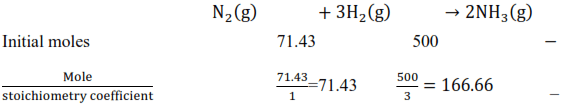From the equation, $1 \mathrm{~mol}$ of nitrogen reacts with $3 \mathrm{~mol}$ of dihydrogen to give 2 moles of ammonia.

$\frac{\text { Mole }}{\text { stoichiometry coefficient }}$, the ratio is less for $\mathrm{N}_{2}$ Hence, $\mathrm{N}_{2}$ is the limiting reagent.
Hence, the moles of ammonia produced by

$71.43$ moles of $\mathrm{N}_{2}=2 \times 71.43$

mass of ammonia produced by

$71.43$ moles of $\mathrm{N}_{2}=2 \times 71.43 \times$

molecular weight of ammonia

$=2 \times 71.43 \times 17 \mathrm{~g}$

$=2428.62 \mathrm{~g}$

(ii) $\mathrm{N}_{2}$ is the limiting reagent and $\mathrm{H}_{2}$ is the excess reagent. Hence, $\mathrm{H}_{2}$ will remain unreacted.

(iii) moles of dihydrogen left unreacted

$=(500-71.43 \times 3)$ moles

Mass of dihydrogen left unreacted

$=(500-71.43 \times 3) 2 g=285.71 \times 2 \mathrm{~g}=571.4 \mathrm{~g}$

Question 25. How are $0.50 \mathrm{~mol} \mathrm{Na}_{2} \mathrm{CO}_{3}$ and $0.50 \mathrm{M} \mathrm{Na}_{2} \mathrm{CO}_{3}$ different?

Solution. Given: mols of $\mathrm{Na}_{2} \mathrm{CO}_{3}=0.50$

Molarity of $\mathrm{Na}_{2} \mathrm{CO}_{3}=0.50 \mathrm{M}$

We know : Molar mass of $\mathrm{Na}_{2} \mathrm{CO}_{3}$

$=(2 \times 23)+12.00+(3 \times 16)$

$=106 \mathrm{gmol}^{-1}$

Now, 1 mole of $\mathrm{Na}_{2} \mathrm{CO}_{3}$ means $106 \mathrm{~g}$ of $\mathrm{Na}_{2} \mathrm{CO}_{3}$

$\therefore 0.5 \mathrm{~mol}$ of $\mathrm{Na}_{2} \mathrm{CO}_{3}$

$=\frac{106 \mathrm{~g}}{1 \mathrm{~mole}} \times 0.5 \mathrm{molNa}_{2} \mathrm{CO}_{3}$

$=53 \mathrm{~g} \mathrm{Na}_{2} \mathrm{CO}_{3}$

$\Rightarrow 0.50 \mathrm{M}$ of $\mathrm{Na}_{2} \mathrm{CO}_{3}=0.50 \frac{\mathrm{mol}}{\mathrm{L}} \mathrm{Na}_{2} \mathrm{CO}_{3}$

Hence, $0.50$ mol of $\mathrm{Na}_{2} \mathrm{CO}_{3}$ is present in $1 \mathrm{~L}$ of water

or $(0.5 \times 106)=53 \mathrm{~g}$ of $\mathrm{Na}_{2} \mathrm{CO}_{3}$ is present in $1 \mathrm{~L}$ of water.

Question 26. If 10 volumes of dihydrogen gas reacts with five volumes of dioxygen gas, how many volumes of water vapour would be produced?

Solution. Reaction of dihydrogen with dioxygen can be written as: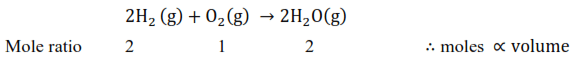According to stiochimerty of reaction two volumes of dihydrogen react with one volume

of dioxygen to produce two volumes of water vapour. Hence, ten volumes of dihydrogen

will react with five volumes of dioxygen to produce ten volumes of water vapour.

Question 27. Convert the following into basic units:

(i) $28.7 \mathrm{pm}$

(ii) $15.15 \mathrm{pm}$

(iii) $25365 \mathrm{mg}$

Solution: (i) $28.7 \mathrm{pm}$ :

$1 \mathrm{pm}=10^{-12} \mathrm{~m}$

$\therefore 28.7 \mathrm{pm}=28.7 \times 10^{-12} \mathrm{~m}$

$=2.87 \times 10^{-11} \mathrm{~m}$

(ii) $15.15 \mathrm{pm}$ :

$1 \mathrm{pm}=10^{-12} \mathrm{~m}$

$\therefore 15.15 \mathrm{pm}=15.15 \times 10^{-12} \mathrm{~m}$

$=1.515 \times 10^{-12} \mathrm{~m}$

(iii) $25365 \mathrm{mg}$ :

$1 \mathrm{mg}=10^{-3} \mathrm{~g}$

$25365 \mathrm{mg}=2.5365 \times 10^{4} \times 10^{-3} \mathrm{~g}$

Since,

$1 \mathrm{~g}=10^{-3} \mathrm{~kg}$

$2.5365 \times 10^{1} \mathrm{~g}=2.5365 \times 10^{-1} \times 10^{-3} \mathrm{~kg}$

$\therefore 25365 \mathrm{mg}=2.5365 \times 10^{-2} \mathrm{~kg}$

Question 28. Which one of the following will have the largest number of atoms?

(i) $1 \mathrm{~g} \mathrm{Au}(\mathrm{s})$

(ii) $1 \mathrm{~g} \mathrm{Na}(\mathrm{s})$

(iii) $1 \mathrm{~g} \mathrm{Li}(\mathrm{s})$

(iv) $1 \mathrm{~g} \mathrm{Cl}_{2}(\mathrm{~g})$

Solution: we know : $\mathrm{N}_{\mathrm{A}}=6.022 \times 10^{23}$

Atomic mass of $\mathrm{Au}=107$

Atomic mass of $\mathrm{Na}=23$

Atomic mass of $\mathrm{Li}=7$

Molar mass of $\mathrm{Cl}_{2}$ molecule $=$ Atomic mass of chlorine

$\times 2=35.5 \times 2=71 \mathrm{gmol}^{-1}$

(i) $1 \mathrm{~g} \mathrm{Au}(\mathrm{s})=\frac{1}{197} \mathrm{~mol}$ of $\mathrm{Au}(\mathrm{s})$

$\frac{1}{197} \times \mathrm{N}_{\mathrm{A}}=\frac{6.022 \times 10^{23}}{197}$ atoms of $\mathrm{Au}(\mathrm{s})$

$=3.06 \times 10^{21}$ atoms of $\mathrm{Au}(\mathrm{s})$

(ii) $1 \mathrm{~g}$ of $\mathrm{Na}(\mathrm{s})=\frac{1}{23}$ mol of $\mathrm{Na}(\mathrm{s})$

$\frac{1}{23} \times \mathrm{N}_{\mathrm{A}}=\frac{6.022 \times 10^{23}}{23}$ atoms of $\mathrm{Na}(\mathrm{s})$

$=0.262 \times 10^{23}$ atoms of $\mathrm{Na}(\mathrm{s})$

$=26.2 \times 10^{21}$ atoms of $\mathrm{Na}(\mathrm{s})$

(iii) 1 g $\mathrm{Li}(\mathrm{s})=\frac{1}{7}$ mol of $\mathrm{Li}(\mathrm{s})$

$=\frac{1}{7} \times \mathrm{N}_{\mathrm{A}}=\frac{6.022 \times 10^{33}}{7}$ atoms of $\mathrm{Li}(\mathrm{s})$

$=0.86 \times 10^{23}$ atoms of $\mathrm{Li}(\mathrm{s})$

$=86.0 \times 10^{21}$ atoms of Li $(s)$

(iv) $1 \mathrm{~g} \mathrm{Cl}_{2}(\mathrm{~g})=\frac{1}{71}$ mol of $\mathrm{Cl}_{2}(\mathrm{~g})$

$=\frac{1}{71} \times \mathrm{N}_{\mathrm{A}}=\frac{6.022 \times 10^{33}}{71}$ atoms of $\mathrm{Cl}_{2}(\mathrm{~g})$

$=0.0848 \times 10^{23}$ atoms of $\mathrm{Cl}_{2}(\mathrm{~g})$\

$=8.48 \times 10^{21}$ atoms of $\mathrm{Cl}_{2}(\mathrm{~g})$

Hence, $1 \mathrm{~g}$ of $\mathrm{Li}(\mathrm{s})$ will have the largest number of atoms.

Question 29. Calculate the molarity of a solution of ethanol in water, in which the mole fraction of ethanol is $0.040$ (assume the density of water to be one).

Solution. Given : mole fraction of ethanol $=0.040$

We know : density of water $=1 \mathrm{gm} / \mathrm{ml}$

the molecular mass of water $\left(\mathrm{H}_{2} \mathrm{O}\right)=18$

Mole fraction of $\mathrm{C}_{2} \mathrm{H}_{5} \mathrm{OH}=\frac{\text { Number of moles of } \mathrm{C}_{2} \mathrm{H}_{5} \mathrm{OH}}{\text { Number of moles of solution }}$

Number of moles of solution

$=$ Number of moles of solute $+$ Number of moles of solvent

Number of moles of solute $=\mathrm{n}_{\mathrm{C}_{2} \mathrm{H}_{5} \mathrm{OH}}$

Number of moles of solvent

$=\mathrm{n}_{\mathrm{H}_{2} \mathrm{O}}=\frac{1000 \mathrm{~g}}{18 \mathrm{gmol}^{-1}}=55.55 \mathrm{~mol}$

$0.040=\frac{\mathrm{n}_{\mathrm{C}_{2} \mathrm{H}_{5} \mathrm{OH}}}{\mathrm{n}_{\mathrm{C}_{2} \mathrm{H}_{5} \mathrm{OH}}+\mathrm{n}_{\mathrm{H}_{2} \mathrm{O}}}$$\ldots(1)$

Substituting the value of $\mathrm{n}_{\mathrm{H}_{2} \mathrm{O}}$ in equation (1),

$\frac{\mathrm{n}_{\mathrm{C}_{2} \mathrm{H}_{5} \mathrm{OH}}}{\mathrm{n}_{\mathrm{C}_{2} \mathrm{H}_{5} \mathrm{OH}}+55.55}=0.040$

$\mathrm{n}_{\mathrm{C}_{2} \mathrm{H}_{5} \mathrm{OH}}=0.040 \mathrm{n}_{\mathrm{C}_{2} \mathrm{H}_{5} \mathrm{OH}}$

$+(0.040) \times(55.55)$

$0.96 \mathrm{n}_{\mathrm{C}_{2} \mathrm{H}_{5} \mathrm{OH}}=2.222 \mathrm{~mol}$

$\mathrm{n}_{\mathrm{C}_{2} \mathrm{H}_{5} \mathrm{OH}}=\frac{2.222}{0.96} \mathrm{~mol}$

$\mathrm{n}_{\mathrm{C}_{2} \mathrm{H}_{5} \mathrm{OH}} \equiv 2.314 \mathrm{~mol}$

Molarity $=\frac{\text { moles of solute }}{\text { volume of solution (liter) }}$

Molarity of solution $=\frac{2.314 \mathrm{mo1}}{1 \mathrm{~L}}$

$=2.314 \mathrm{M}$

Question 30. What will be the mass of one ${ }^{12} \mathrm{C}$ atom in g?

Solution. 1 mole of carbon atoms $=6.023 \times 10^{23}$ atoms of carbon

$=12 \mathrm{~g}$ of carbon

$\therefore$ Mass of one ${ }^{12} \mathrm{C}=12 \times 1.67 \times 10^{-24} \mathrm{~g}$

$=1.993 \times 10^{-23} \mathrm{~g}$

Question 31. How many significant figures should be present in the answer of the following calculations?

(i) $\frac{0.02856 \times 298.15 \times 0.112}{0.5785}$

(ii) $5 \times 5.364$

(iii) $0.0125+0.7864+0.0215$

Solution. (i) $\frac{0.02856 \times 298.15 \times 0.112}{0.5785}$

Least precise number of calculation $=0.112$

$\therefore$ Number of significant figures in the answer.

$=$ Number of significant figures in the least precise number.

$=3$

(ii) $5 \times 5.364$

Least precise number of calculation $=5.364$

$\therefore$ Number of significant figures in the answer $=$ Number of significant figures in $5.364$

$=4$

(iii) $0.0125+0.7864+0.0215$

Since the least number of decimal places in each term is four, the number of significant figures in the answer is also $4 .$

Question 32. Use the data given in the following table to calculate the molar mass of naturally occuring argon isotopes:

Solution.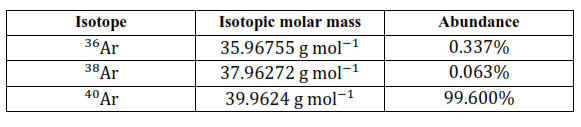Given :

for Isotope ${ }^{36} \mathrm{Ar}$ :Isotopic molar mass

$35.96755 \mathrm{~g} \mathrm{~mol}^{-1}$, percentage Abundance $0.337 \%$

for Isotope ${ }^{38} \mathrm{Ar}:$

Isotopic molar mass $37.96272 \mathrm{~g} \mathrm{~mol}^{-1}$ percentage Abundance $0.063 \%$

for Isotope

${ }^{40} \mathrm{Ar}: \quad$ Isotopic molar mass $39.9624 \mathrm{~g} \mathrm{~mol}^{-1}$
percentage Abundance99.600\%

Average molar mass

$=$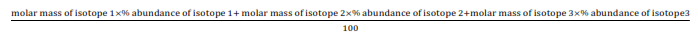Average molar mass of argon

$=\left[\left(35.96755 \times \frac{0.337}{100}\right)+\left(37.96272 \times \frac{0.063}{100}\right)\right.$

$\left.+\left(39.9624 \times \frac{90.60}{100}\right)\right] \mathrm{gmo1}^{-1}$

$=[0.121+0.024+39.802] \mathrm{gmo} 1^{-1}$

$=39.947 \mathrm{~g} \mathrm{~mol}^{-1}$

Question 33. Calculate the number of atoms in each of the following

(i) 52 moles of $\mathrm{Ar}$

(ii) $52 \mathrm{u}$ of $\mathrm{He}$

(iii) $52 \mathrm{~g}$ of $\mathrm{He}$.

Solution: We know : atomic mass of $\mathrm{He}=4 ; \mathrm{N}_{\mathrm{A}}=6.022 \times 10^{23}$

(i) 1 mole of $\mathrm{Ar}=\mathrm{N}_{\mathrm{A}}$ atoms of $\mathrm{Ar}$

$=6.022 \times 10^{23}$ atoms of $\mathrm{Ar}$

$\therefore 52$ mol of $\mathrm{Ar}=52 \times 6.022 \times 10^{23}$ atoms of $\mathrm{Ar}$

$=3.131 \times 10^{25}$ atoms of $\mathrm{Ar}$

(ii) 1 atom of $\mathrm{He}=4 \mathrm{u}$ of $\mathrm{He}$

Or,

$4 \mathrm{u}$ of $\mathrm{He}=1$ atom of $\mathrm{He}$

$1 \mathrm{u}$ of $\mathrm{He}=\frac{1}{4}$ atom of $\mathrm{He}$

$52 \mathrm{u}$ of $\mathrm{He}=\frac{52}{4} u$ atom of $\mathrm{He}$

$=13 \mathrm{u}$ atoms of $\mathrm{He}$

$=13 \mathrm{u}$ atoms of $\mathrm{He}$

(ii) I mole of He atom $=4 \mathrm{~g} \mathrm{He}$

I mole of He atom $=N_{A}$ atoms of He

$=6.022 \times 10^{23}$ atoms of $\mathrm{He}$

So $4 \mathrm{~g}$ of $\mathrm{He}=6.022 \times 10^{23}$ atoms of $\mathrm{He}$

$\therefore 52 \mathrm{~g}$ of $\mathrm{He}=\frac{6.022 \times 10^{23} \times 52}{4}$ atoms of $\mathrm{He}$

$=7.8286 \times 10^{24}$ atoms of $\mathrm{He}$

Question 34. A welding fuel gas contains carbon and hydrogen only. Burning a small sample of it in oxygen gives $3.38 \mathrm{~g}$ carbon dioxide, $0.690 \mathrm{~g}$ of water and no other products. A volume of $10.0 \mathrm{~L}$ (measured at STP) of this welding gas is found to weigh $11.6 \mathrm{~g}$. Calculate

(i) empirical formula,

(ii) molar mass of the gas, and

(iii) molecular formula.

Solution:

Given: a mass of carbon dioxide in welding fuel gas $=3.38 \mathrm{~g}$

Mass of Water welding fuel gas $=0.690 \mathrm{~g}$

The volume of welding fuel gas $=10$ litre

Weight od welding fuel gas $=11.6 \mathrm{~g}$

(i) We know 1 mole $(44 \mathrm{~g})$ of $\mathrm{CO}_{2}$ contains $12 \mathrm{~g}$ of carbon.

$\therefore 3.38 \mathrm{~g}$ of $\mathrm{CO}_{2}$ will contain carbon

$=\frac{12 \mathrm{~g}}{44 \mathrm{~g}} \times 3.38 \mathrm{~g}$

$=0.9217 \mathrm{~g}$

we know $18 \mathrm{~g}$ of water $\left(\mathrm{H}_{2} \mathrm{O}\right)$ contains $2 \mathrm{~g}$ of hydrogen.

$\therefore 0.690 \mathrm{~g}$ of water will contain hydrogen

$=\frac{2 \mathrm{~g}}{18 \mathrm{~g}} \times 0.690$

$=0.0767 \mathrm{~g}$

Since carbon and hydrogen are the only constituents of the compound, the total mass of

the compound is:

$=0.9217 \mathrm{~g}+0.0767 \mathrm{~g}$

$=0.9984 \mathrm{~g}$

Formula: Percent of atom in the compound

$=\frac{\text { weight of atom }}{\text { weight of sample }} \times 100$

$\therefore$ Percent of $C$ in the compound

$=\frac{0.9217 \mathrm{~g}}{0.9984 \mathrm{~g}} \times 100$

$=92.32 \%$

Percent of $\mathrm{H}$ in the compound

$=\frac{0.0767 \mathrm{~g}}{0.9984 \mathrm{~g}} \times 100$

$=7.68 \%$

Formula : moles $=\frac{\text { weight }}{\text { atomic weight }}$

Moles of carbon in the compound $=\frac{92.32}{12}$

$=7.69$

Moles of hydrogen in the compound $=\frac{7.68}{1}$

$=7.68$

$\therefore$ Ratio of carbon to hydrogen in the compound $=7.69: 7.68$

$=1: 1$

Hence, the empirical formula of the gas is $\mathrm{CH}$.

(ii) Given,

Weight of $10.0 \mathrm{~L}$ of the gas (at S.T.P) $=11.6 \mathrm{~g}$

$\therefore$ Weight of $22.4 \mathrm{~L}$ of gas at $\mathrm{STP}=\frac{11.6 \mathrm{~g}}{10 \mathrm{~L}} \times 22.4 \mathrm{~L}$

$=25.984 \mathrm{~g}$

$\approx 26 g$

Hence the molar mass of the gas is $26 \mathrm{~g}$.

(iii) Empirical formula mass of $\mathrm{CH}=12+1=13 \mathrm{~g}$

$\mathrm{n}=\frac{\text { Molar mass of gas }}{\text { Empirical formula mass of gas }}$

$=\frac{26 g}{13 g}$

$\mathrm{n}=2$

formula : Molecular formula of gas $=($ emperical formula of gas $) \times n \mathrm{n}$

$\therefore$ Molecular formula of gas $=(\mathrm{CH})_{n}$

$=\mathrm{C}_{2} \mathrm{H}_{2}$

Question 35. Calcium carbonate reacts with aqueous $\mathrm{HCl}$ to give $\mathrm{CaCl}_{2}$ and $\mathrm{CO}_{2}$ according to the reaction,

$\mathrm{CaCO}_{3}(\mathrm{~s})+2 \mathrm{HCl}(\mathrm{aq}) \rightarrow \mathrm{CaCl}_{2}(\mathrm{aq})$

$+\mathrm{CO}_{2}(\mathrm{~g})+\mathrm{H}_{2} \mathrm{O}(\mathrm{l})$

What mass of $\mathrm{CaCO}_{3}$ is required to react completely with $25 \mathrm{~mL}$ of $0.75 \mathrm{M} \mathrm{HCl}$ ?

Solution: given : volume of $\mathrm{HCl}$ solution $=25 \mathrm{~mL}$

molarity of $\mathrm{HCl}$ solution $=0.75 \mathrm{M}$

we know molecular formula of $\mathrm{CaCO}_{3}=100$

formula : moles $=$ molarity $\times$ volume (litre)

moles of $\mathrm{HCl}=\frac{0.75 \mathrm{M} \times 25 \mathrm{~mL}}{1000}=0.01875$

From the given chemical equation,

$\mathrm{CaCO}_{3}(\mathrm{~s})+2 \mathrm{HCl}(\mathrm{aq})$

$\rightarrow \mathrm{CaCl}_{2}(\mathrm{aq})+\mathrm{CO}_{2}(\mathrm{~g})+\mathrm{H}_{2} \mathrm{O}(\mathrm{l})$

According to stoichiometry of reaction :

$\therefore 2 \mathrm{~mol}$ of $\mathrm{HCl}$ react with $=1 \mathrm{~mol}$ of $\mathrm{CaCO}_{3}$

$0.01875 \mathrm{~mol} \mathrm{HCl}$ of react with

$=\frac{1}{2} \times 0.01875 \mathrm{~mol}$ of $\mathrm{CaCO}_{3}$

$(\therefore$ weight $=$ moles $\times$ molecular weight $)$

Amount of $\mathrm{CaCO}_{3}$ that will react

$=\frac{1}{2} \times 0.01875 \times 100$

$=0.9375 \mathrm{~g}$

Question 36. Chlorine is prepared in the laboratory by treating manganese dioxide $\left(\mathrm{MnO}_{2}\right)$ with

aqueous hydrochloric acid according to the reaction

$4 \mathrm{HCl}(\mathrm{aq})+\mathrm{MnO}_{2}(\mathrm{~s})$

$\rightarrow 2 \mathrm{H}_{2} \mathrm{O}(\mathrm{l})+\mathrm{MnCl}_{2}(\mathrm{aq})+\mathrm{Cl}_{2}(\mathrm{~g})$

How many grams of HCl react with $5.0 \mathrm{~g}$ of manganese dioxide?

Solution: given : weight of manganese dioxide $=5 \mathrm{~g}$

we know molecular mass of $\mathrm{Mn} \mathrm{O}_{2}$

$=[55+2 \times 16=87 g]$

moles of $\mathrm{MnO}_{2}=\frac{\text { weight }}{\text { molecular weight }}$

$=\frac{5}{87}=0.057 \mathrm{~mole}$

according to stoichiometry

1 mole $\mathrm{MnO}_{2}$ reacts completely with $4 \mathrm{~mol}$ of $\mathrm{HCl}$.

$\therefore 0.057 \mathrm{~mole}$ of $\mathrm{MnO}_{2}$ will react with $\mathrm{HCl}$

$=\frac{4}{1} \times 0.057=$

$0.2298$ mole of HCl formed

weight of $\mathrm{HCl}=$ moles molecular

$\times$ moles of $\mathrm{HCl}=36.5 \times 0.2298=8.4 \mathrm{~g}$

Hence, $8.4 \mathrm{~g}$ of $\mathrm{HCl}$ will react completely with $5.0 \mathrm{~g}$ of manganese dioxide.